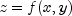JMSLTM Numerical Library 7.2.0
com.imsl.chart3d

Class Surface

• Field Detail

• SURFACE_TYPE_FLAT

public static final int SURFACE_TYPE_FLAT
Draws the surface using flat shading.

In a flat shaded surface, each polygon has a uniform color.

Constant Field Values
• SURFACE_TYPE_GOURAUD

public static final int SURFACE_TYPE_GOURAUD
Draws the surface using Gouraud shading. In a Gouraud shaded surface, colors are interpolated across each polygon.
Constant Field Values
• SURFACE_TYPE_MESH

public static final int SURFACE_TYPE_MESH
Draws the surface as a mesh.
Constant Field Values
• SURFACE_TYPE_NICEST

public static final int SURFACE_TYPE_NICEST
Draws the surface using the best shading available.
Constant Field Values
• Constructor Detail

• Surface

public Surface(AxisXYZ parent,
double[] x,
double[] y,
double[] z)
Creates a surface from a scattered set of 3D points.

A surface is created from a scattered set of points. A function is interpolated to the given point over the rectangular area determined by that point's ranges.

Parameters:
parent - an AxisXYZ object, the parent of this node.
x - is the array of x values.
y - is the array of y values.
z - is the array of z values.
• Surface

public Surface(AxisXYZ parent,
double[] x,
double[] y,
double[][] z)
Creates a surface from a gridded data set. A surface is created from a grid of points in a rectangular area. The point z[i][j] is the z-value at (x[i], y[j]).
Parameters:
parent - an AxisXYZ object, the parent of this node.
x - is the array of x values.
y - is the array of y values.
z - is the two-dimensional array of z values of size x.length by y.length.
• Surface

public Surface(AxisXYZ parent,
double[] x,
double[] y,
double[][] z,
Color[][] color)
Creates a colored surface from a gridded data set.

A surface is created from a grid of points in a rectangular area. The point z[i][j] is the z-value at (x[i], y[j]).

Parameters:
parent - an AxisXYZ object, the parent of this node.
x - is the array of x values.
y - is the array of y values.
z - is the two-dimensional array of z values.
color - is the two-dimensional array of color values. The array must have the same size as the array z.
• Surface

public Surface(AxisXYZ parent,
double[] x,
double[] y,
double[] z,
Color[] color)
Creates a surface from a scattered set of 3D points with a color given at each point.

A surface is created from a scattered set of points. A function is interpolated to the given point over the rectangular area determined by that point's ranges.

Parameters:
parent - an AxisXYZ object, the parent of this node.
x - is the array of x values.
y - is the array of y values.
z - is the array of z values.
color - is array of color values at each point.
• Surface

public Surface(AxisXYZ parent,
Surface.ZFunction zFunction,
double xmin,
double xmax,
double ymin,
double ymax)
Creates a surface from a function. A surface is created by evaluation of the function on a grid of points in a rectangular area, [xmin,xmax] by [ymin,ymax], of the xy-plane.
Parameters:
parent - an AxisXYZ object, the parent of this node.
zFunction - the function,.
xmin - the minimum x-value of the function rectangle.
xmax - the maximum x-value of the function rectangle.
ymin - the minimum y-value of the function rectangle.
ymax - the maximum y-value of the function rectangle.
• Method Detail

Description copied from class: ChartNode3D
Called to add this object to the scene graph.
Overrides:
Parameters:
parent - is the node in the scene graph at which this object is to be added.
• dataRange

public void dataRange(double[] range)
Update the data range, range = {xmin,xmax,ymin,ymax}. The entries in range are updated to reflect the extent of the data in this node. The argument range is an input/output variable. Its value should be updated only if the data in this node is outside the range already in the array.
Overrides:
dataRange in class Data
Parameters:
range - a double array which contains the updated range, {xmin,xmax,ymin,ymax}
• getNumberGridPointsX

public int getNumberGridPointsX()
Returns the value of the "NumberGridPointsX" attribute.

This is the grid points in the x-direction for surfaces defined by a function.

Returns:
The number of grid points in the x-direction. Default is 40.
• getNumberGridPointsY

public int getNumberGridPointsY()
Returns the value of the "NumberGridPointsY" attribute.

This is the grid points in the y-direction for surfaces defined by a function.

Returns:
The number of grid points in the y-direction. Default is 40.
• getSurfaceType

public int getSurfaceType()
Returns the attribute "SurfaceType".
Returns:
one of SURFACE_TYPE_MESH, SURFACE_TYPE_FLAT, SURFACE_TYPE_GOURAUD, SURFACE_TYPE_NICEST or SURFACE_TYPE_MESH or-ed with one of the other types. Default value is SURFACE_TYPE_NICEST.
SURFACE_TYPE_MESH, SURFACE_TYPE_FLAT, SURFACE_TYPE_GOURAUD, SURFACE_TYPE_NICEST
• setNumberGridPointsX

public void setNumberGridPointsX(int nx)
Sets the value of the "NumberGridPointsX" attribute.

This is the grid points in the x-direction for surfaces defined by a function.

Parameters:
nx - The number of grid points in the x-direction. Default is 40.
• setNumberGridPointsY

public void setNumberGridPointsY(int ny)
Sets the value of the "NumberGridPointsY" attribute.

This is the grid points in the y-direction for surfaces defined by a function.

Parameters:
ny - The number of grid points in the y-direction. Default is 40.
• setSurfaceType

public void setSurfaceType(int surfaceType)
Sets the attribute "SurfaceType".
Parameters:
surfaceType - is one of SURFACE_TYPE_MESH, SURFACE_TYPE_FLAT, SURFACE_TYPE_GOURAUD, SURFACE_TYPE_NICEST or SURFACE_TYPE_MESH or-ed with one of the other types.